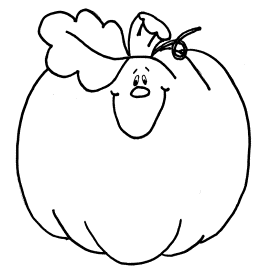# Autumn Themed Math Pages

Always remember that grade levels are not absolutes -- especially since we're all living in different countries and therefore have different curriculums.  Some kindergarten children will find the Grade One worksheets useful as will some Grade Two students.  Use your judgment!NOTE:  Magic Squares definition:  add across and down to see the relationships in addition and subtraction.  Great self-checking review (the sums of the answers across = the sums of the answers down making it possible for the children to check their own answers)

1. Two numbers from 0 to 10
2. Addition (single digit) - Autumn/Nature theme activity worksheet
3. Subtraction (single digit) - Autumn/Nature theme activity worksheet
4. Fill in the blanks - two numbers from 0 to 10
5. Add a column of three numbers (the first number is always "1")
6. One number from 10 to 19 and one number from 0 to 9   (addition)   (subtraction)
7. Single digit number MAGIC SQUARES  (addition)   (subtraction)
8. Single digit number MATH TABLES  (addition)   (subtraction
9. Single digit number sentences - fill in the + or - sign (addition/subtraction
10. Word Problems

1. One number from 10 to 99 and one number from 0 to 9   (addition)   (subtraction)
2. Number sentences - fill in the + or - sign (addition/subtraction
3. Two digit numbers - NO CARRYING   (addition)   (subtraction
4. Two digit numbers - CARRYING
5. Fill in the blanks - two digit numbers with CARRYING
6. Two digit number MAGIC SQUARES   (addition)   (subtraction
7. Two digit number MATH TABLES  (addition)   (subtraction
8. Two digit number sentences - fill in the + or - sign (addition/subtraction
9. Add a column of three single digit numbers
10. Two numbers from 0 to 10   (multiplication)
11. Multiplication MATH TABLES  (multiplication)
12. Number sentences - fill in the + or - or x sign (addition/subtraction/multiplication
13. Word Problems

1. Three digit number   (addition)   (subtraction
2. Fill in the blanks - three digit number   (addition)   (subtraction)
3. Three digit number MAGIC SQUARES   (addition)   (subtraction)
4. Three digit number MATH TABLES  (addition)   (subtraction
5. Add a column of three double digit numbers
6. Four digit number   (addition)   (subtraction
7. Fill in the blanks - four digit number   (addition)   (subtraction)
8. Four digit number MAGIC SQUARES   (addition)   (subtraction)
9. Four digit number MATH TABLES  (addition)   (subtraction
10. Decimal numbers - NO CARRYING   (addition)   (subtraction)
11. Decimal numbers - CARRYING   (addition)   (subtraction)
12. Multiply one number from 10 to 100 and one number from 0 to 10
13. Find the missing factor (1 to 10)  (vertical)   (horizontal
14. Division - single digit quotient without remainders
15. Division - double digit quotient without remainders
16. Word Problems

1. Five digit number   (addition)   (subtraction
2. Fill in the blanks - five digit number   (addition)   (subtraction)
3. Two digit decimal numbers   (addition)   (subtraction)
4. Add a column of 3 two digit decimal numbers
5. Multiply two digit numbers
6. Find the missing factor (1 to 100)  (vertical)   (horizontal
7. Two digit multiplication MATH TABLES  (multiplication)
8. Division - triple digit quotient without remainders
9. Division - Two digit division with remainders
10. Word Problems

1. Multiply three digit numbers
2. Three digit multiplication MATH TABLES  (multiplication)
3. Four digit multiplication MATH TABLES  (multiplication)
4. Multiply a decimal number by a whole number
5. Divide a decimal number by a whole number
6. Multiply decimal numbers
7. Word Problems

## Directions:

For static math worksheets click one of the links above, click on the template image to open it in a new window and use the print function of your browser.

For dynamic math worksheets the link above will open in a new window. Use the "PRINT" link near the top of the page to print the worksheet. To generate a new worksheet simply refresh or reload the page in your browser.